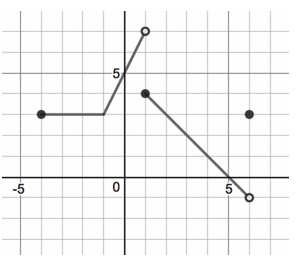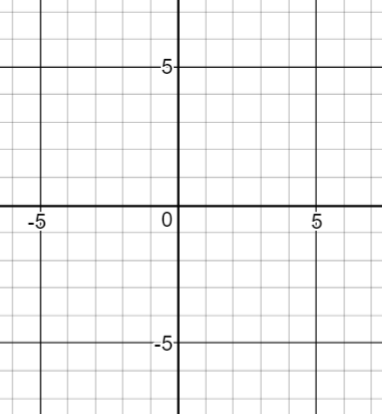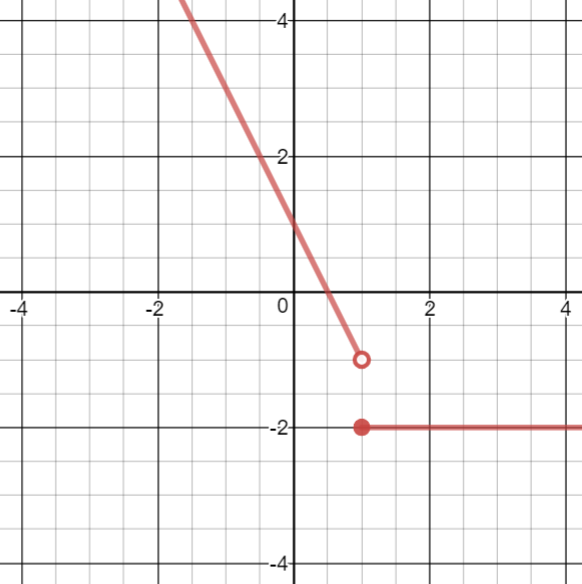Match Fishtank is now Fishtank Learning!

# Limits and Continuity

## Objective

Graph, write, and evaluate linear piecewise functions.

## Criteria for Success

?

1. Describe that a piecewise function is a function that is defined differently on distinct domains.
2. Write equations and domain restrictions for parts of a piecewise function.
3. Identify and graph endpoints of open and closed intervals.
4. Sketch linear functions on a specified domain.

## Tips for Teachers

?

Desmos has a tutorial, Learn Desmos: Restrictions, on creating graphs with domain or range restrictions. Take a look at this before creating the problem set and note that open and closed points must be added separately from the functions.

## Anchor Problems

?

### Problem 1

Write the piecewise function shown in the graph below.### Problem 2

Graph the following piecewise function. Describe whether the function is continuous or discontinuous.

$f(x)=\left\{\begin{matrix} -{1\over2}x+4, \space x>2 \\ -2x+1, \space \space \space -2<x\leq2 \end{matrix}\right.$

## Problem Set

?

The following resources include problems and activities aligned to the objective of the lesson that can be used to create your own problem set.

• Include problems where functions:
• Have a break on the ${{y-}}$axis
• Have a break on a value other than the ${{y-}}$axis
• Have a mix of negative, positive and zero slopes
• Error analysis where the domain restrictions are not applied appropriately and the graph is not a function (both graphically and algebraically)
• (This activity can be copied and revised to just be linear functions, or you can use it with non-linear for JUST the domain restrictions on pieces.)
• Kuta Software Free Precalculus Worksheets Free Precalculus WorksheetsAny in the "Functions" section

?

### Problem 1

Graph the piecewise function.${f(x)=\left\{\begin{matrix}x-4, \space \mathrm{if}\space x\leq1 \\ 3x,\space \mathrm{if}\space x>1 \end{matrix}\right.}$

### Problem 2

Write equations for the piecewise function shown below.